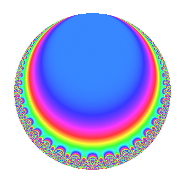Properties

 Label 128.5.d.bLevel 128 Weight 5 Character orbit 128.d Self dual Yes Analytic conductor 13.231 Analytic rank 0 Dimension 2 CM disc. -8 Inner twists 4

Related objects

Newspace parameters

 Level: $$N$$ = $$128 = 2^{7}$$ Weight: $$k$$ = $$5$$ Character orbit: $$[\chi]$$ = 128.d (of order $$2$$ and degree $$1$$)

Newform invariants

 Self dual: Yes Analytic conductor: $$13.2313552747$$ Analytic rank: $$0$$ Dimension: $$2$$ Coefficient field: $$\Q(\sqrt{2})$$ Coefficient ring: $$\Z[a_1, a_2, a_3]$$ Coefficient ring index: $$2^{3}$$ Sato-Tate group: $\mathrm{U}(1)[D_{2}]$

$q$-expansion

Coefficients of the $$q$$-expansion are expressed in terms of $$\beta = 8\sqrt{2}$$. We also show the integral $$q$$-expansion of the trace form.

 $$f(q)$$ $$=$$ $$q$$ $$+ \beta q^{3}$$ $$+ 47 q^{9}$$ $$+O(q^{10})$$ $$q$$ $$+ \beta q^{3}$$ $$+ 47 q^{9}$$ $$+ 21 \beta q^{11}$$ $$+ 574 q^{17}$$ $$-51 \beta q^{19}$$ $$+ 625 q^{25}$$ $$-34 \beta q^{27}$$ $$+ 2688 q^{33}$$ $$-1246 q^{41}$$ $$+ 105 \beta q^{43}$$ $$+ 2401 q^{49}$$ $$+ 574 \beta q^{51}$$ $$-6528 q^{57}$$ $$-615 \beta q^{59}$$ $$-651 \beta q^{67}$$ $$-9506 q^{73}$$ $$+ 625 \beta q^{75}$$ $$-8159 q^{81}$$ $$-711 \beta q^{83}$$ $$-5474 q^{89}$$ $$+ 9982 q^{97}$$ $$+ 987 \beta q^{99}$$ $$+O(q^{100})$$ $$\operatorname{Tr}(f)(q)$$ $$=$$ $$2q$$ $$\mathstrut +\mathstrut 94q^{9}$$ $$\mathstrut +\mathstrut O(q^{10})$$ $$2q$$ $$\mathstrut +\mathstrut 94q^{9}$$ $$\mathstrut +\mathstrut 1148q^{17}$$ $$\mathstrut +\mathstrut 1250q^{25}$$ $$\mathstrut +\mathstrut 5376q^{33}$$ $$\mathstrut -\mathstrut 2492q^{41}$$ $$\mathstrut +\mathstrut 4802q^{49}$$ $$\mathstrut -\mathstrut 13056q^{57}$$ $$\mathstrut -\mathstrut 19012q^{73}$$ $$\mathstrut -\mathstrut 16318q^{81}$$ $$\mathstrut -\mathstrut 10948q^{89}$$ $$\mathstrut +\mathstrut 19964q^{97}$$ $$\mathstrut +\mathstrut O(q^{100})$$

Character Values

We give the values of $$\chi$$ on generators for $$\left(\mathbb{Z}/128\mathbb{Z}\right)^\times$$.

 $$n$$ $$5$$ $$127$$ $$\chi(n)$$ $$-1$$ $$-1$$

Embeddings

For each embedding $$\iota_m$$ of the coefficient field, the values $$\iota_m(a_n)$$ are shown below.

For more information on an embedded modular form you can click on its label.

Label $$\iota_m(\nu)$$ $$a_{2}$$ $$a_{3}$$ $$a_{4}$$ $$a_{5}$$ $$a_{6}$$ $$a_{7}$$ $$a_{8}$$ $$a_{9}$$ $$a_{10}$$
63.1
 −1.41421 1.41421
0 −11.3137 0 0 0 0 0 47.0000 0
63.2 0 11.3137 0 0 0 0 0 47.0000 0
 $$n$$: e.g. 2-40 or 990-1000 Significant digits: Format: Complex embeddings Normalized embeddings Satake parameters Satake angles

Inner twists

Char. orbit Parity Mult. Self Twist Proved
1.a Even 1 trivial yes
8.d Odd 1 CM by $$\Q(\sqrt{-2})$$ yes
4.b Odd 1 yes
8.b Even 1 yes

Hecke kernels

This newform can be constructed as the kernel of the linear operator $$T_{3}^{2}$$ $$\mathstrut -\mathstrut 128$$ acting on $$S_{5}^{\mathrm{new}}(128, [\chi])$$.